# How to interface a 5V output to a 3.3V input.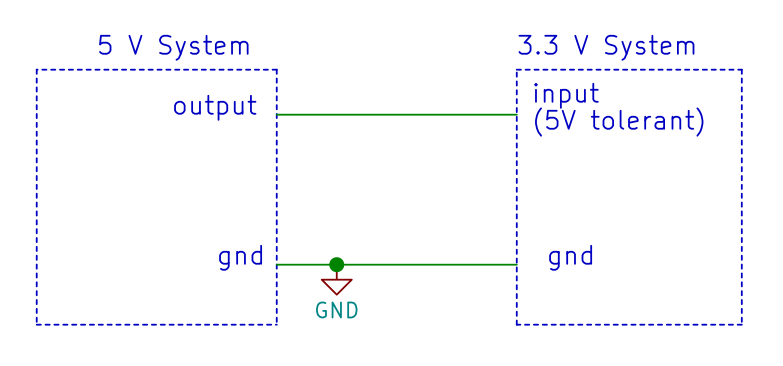Many times we must interface 5V to 3.3V devices. This occurs very frequently on Arduino, but other applications need 5V to 3.3V ICs or systems to communicate.

For instance, imagine you want to connect to your Arduino Uno, which works at 5V, a 3.3V device, such as the display we used in our guide “How to play a video on Arduino Uno (2/6)”. Similarly, there are many 5V devices or shields, that cannot be used with an Arduino 2, which works at 3.3 V.

In this post, we will talk about 5V to 3.3V interfacing. For the opposite task, i.e. 3.3V to 5V conversion, see this article.

There are many ways to perform 5V to 3.3V interfacing. The most common are:

• Direct Connection (Warning: Only With 5V Tolerant Inputs!!!)
• Series resistor.
• Series resistor with external Schottky diode clamp toward the 3.3V line.
• Series diode with pull-up resistor.
• Series resistor with active clamp.
• Resistor and Zener diode.
• Resistor divider.
• BJT/MOSFET as inverters.
• Two cascaded BJT/MOSFET as buffer.
• Pull-up resistor on open drain/collector outputs.
• Adding 5V-powered logic ICs (buffer, gates, etc) with open drain/collector output.
• Adding 3.3V-powered logic ics (buffer, gates, etc) with 5-V tolerant input.
• Series MOSFET (common gate configuration).
• Series BJT (common base configuration).
• Level translator.
• Optocoupler.

## DIRECT CONNECTION (WARNING: ONLY WITH 5V TOLERANT INPUTS!!!)

The simplest way is, a direct connection. This is the case of the Arduino Ethernet Shield: the Wiznet W5500 Ethernet chip works at 3.3V but has 5V tolerant Inputs (see datasheet). The Arduino 2, instead, has NO 5V tolerant input, therefore you should never directly connect a 5V output to any of its input pins!Fig. 1. Direct connection is possible if the 3.3V device has a 5-V tolerant input.

• No additional cost or space.
• Fastest solution.

• It works only on 5V-tolerant devices.

## SERIES RESISTOR

The second simplest way is to use a series resistor.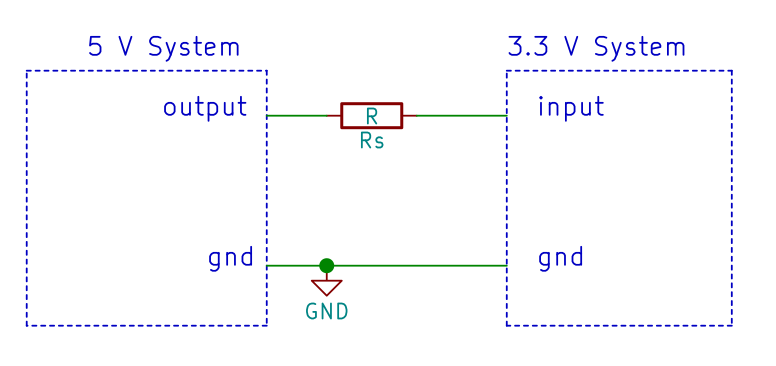Fig. 2. Series resistor used to interface a 5V outputto a 3.3V input. The input must have its protection diodes!

How does it work:

The input of a 3.3V (non 5V tolerant IC) is normally protected by diodes: one is toward VDD, another is toward GND, as shown in the figure below.These diodes are normally OFF as they are both reverse biased. However, if you try to put a voltage larger than VDD,3.3V, they will clamp the input voltage to VDD,3.3V (plus the forward diode voltage, normally 0.7V). Transient spikes larger than VDD,3.3V can occur even on a 3.3V-only system, due to capacitive coupling of switching device, or ringing.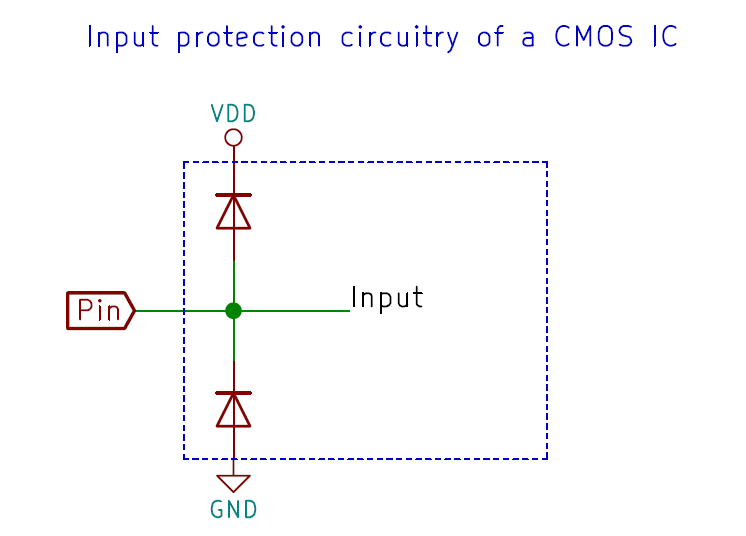Fig. 3. Simplified schematics of the input protection circuitry on a CMOS IC.

When a 5V is fed through the resistor to the input, the diode will turn on: the resistor limits the current that flows into the pin, protecting the input. The maximum current into pin is sometimes indicated in the datasheet, and it should be kept low for two reasons:

• Normally, except the leakage current, no current would flow into a pin. Damage could occur, due to latch-up phenomena.
• The current flowing into the input pin, will flow OUT from the VDD pins! If such current is too large, it could increase the VDD voltage of the whole system, destroying the IC. If the system has a current consumption which is larger than the current flowing into the PIN, then there should be no problems. Otherwise, it’s advisable to put a dummy load, between VDD,3.3V and GND. The value of the load should be so that it adsorbs at least the current flowing into ALL The inputs.

To calculate this, simply consider the input current as I=(5V-3.3V)/RS. Then put between VDD,3.3V and GND a resistor such that it will draw the same current I. In other words: RLOAD = RS 3.3V (5V-3.3V) or about 2 RS. If you have more than one input, RLOAD must be decreased accordingly. If your system already draws “I” from the 3.3V (e.g. due to the IC current consumption, or presence of an always-on LED), then you might omit or increase RLOAD.

This solution has also another drawback: it severely limits the bandwidth, unless low valued resistors are used. In fact, the input pin and the traces will have a certain parasitic capacitance Cparasitic.

The bandwidth of the system is fC=1/(2πRSCparasitic). This does not means that you can hope for a fC digital clock signal to pass without being severely distorted. Here we plot a 500kHz square wave, after a filter, with a 1-MHz fC.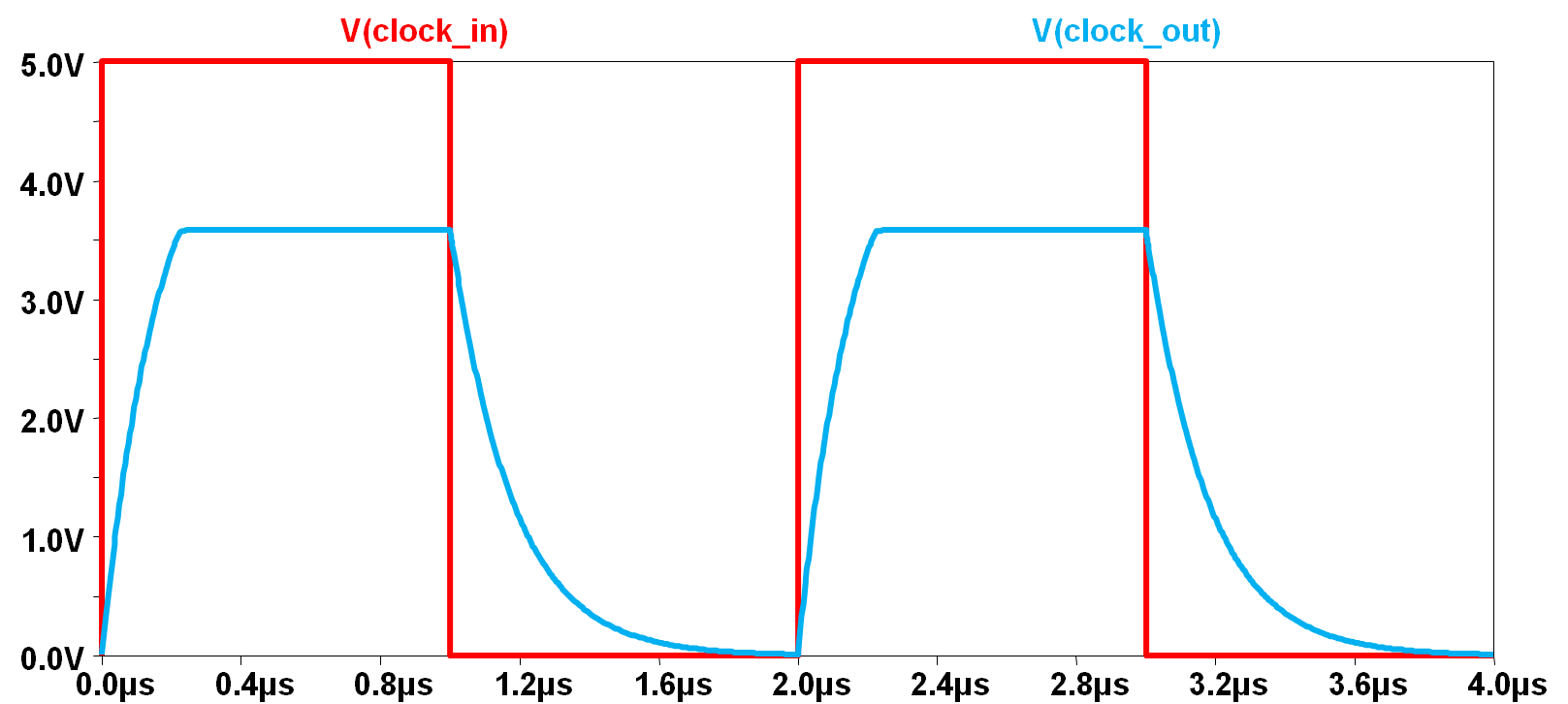Fig. 4. A 500-kHz clock is fed to the circuit of Fig.2 (red line). The blue line is the actual obtained waveform.

To improve the bandwidth, low-value resistors should be used. However, low value resistors are not advisable for these reasons:

• The already mentioned current into pin.
• The current consumption.
• The overload of the output.
• It generates additional noises on the VDD,3.3V line as the input is switched.

Practical values are in the 1-10kOhm.

• Simple solution: just one resistor per input pin.

• High power usage (with low-value resistors) or poor bandwidth (high value resistors).
• Output overload (with low-value resistors)
• The current going into the input might cause latch up.
• Requires an external load resistors on the 3.3V supply, if the 3.3V system has a very low minimum current consumption.
• Possible noise on the 3.3V supply due to current injected into it.
• The 3.3V input must have a high impedance (small input leakage, such as CMOS).

## SERIES RESISTOR WITH EXTERNAL SCHOTTKY DIODE CLAMP TOWARD THE 3.3V LINE.

This circuit is similar to the previous solution, however faster speeds can be achieved, because the majority of the current will be carried by the schottky diode, instead of the protection diodes.

This still has the drawback of having the current being fed to the VDD,3.3V line. Such current must therefore be “consumed” by the system, as shown for the previous case.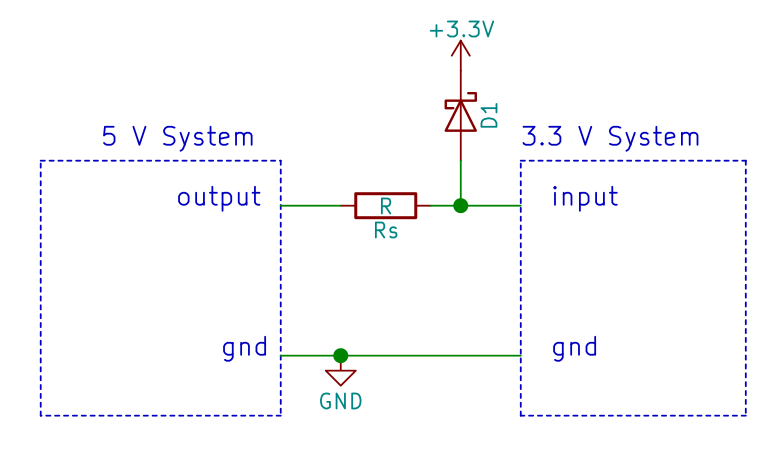Fig. 5. Adding a Schottky diode, allows using smaller resistors, thus improving the bandwidth.

• Larger bandwidth achievable.
• Negligible current injected into input pin.

• Requires two components per input (resistor and Schottky diode).
• High power usage (with low-value resistors) or poor bandwidth (high value resistors).
• Output overload (with low-value resistors)
• Requires an external load resistors on the 3.3V supply, if the 3.3V system has a very low minimum current consumption.
• Possible noise on the 3.3V supply due to current injected into it.
• The capacitance of the diode must be still taken into account.
• The 3.3V input must have a high impedance (small input leakage, such as CMOS).

## SERIES RESISTOR WITH ACTIVE CLAMP.

Instead of redirecting the input current toward VDD,3.3V, it’s better to dissipate it to ground, so no VDD,3.3V level variations will occur.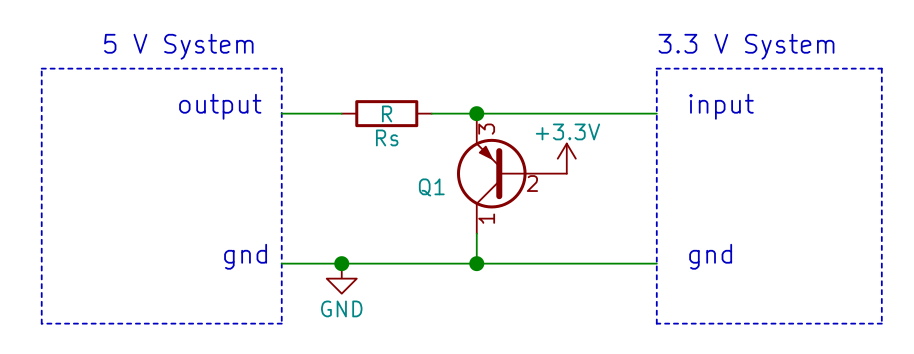Fig. 6. A PNP BJT can be used as active clamp, by shorting to ground (instead of 3.3V) the excess current.

The PNP BJT will conduct as long when the input voltage is larger than 3.9V.
This solution allows using smaller resistors (higher bandwidth).

Noticeably, there will be still current injected into the VDD,3.3V, however, such current will be hFE times smaller than the current flowing into the RS. Therefore, in the majority of the cases, no RLOAD is required.

Another problem is that, on some ICs, their protection diode start conducting when the values on the input is as low as 0.35V above the 3.3V level. In such case, the BJT base should not be tied to 3.3V, but to a somewhat smaller voltage, if available on the system.

• Even larger bandwidth achievable.
• Very small current injected into the 3.3V supply line.
• Small current injected into the input pin.

• Requires two components per input (resistor and BJT).
• High power usage (with low-value resistors) or poor bandwidth (high value resistors).
• Output overload (with low-value resistors)
• Still might require an external load resistors on the 3.3V supply, if the 3.3V system has an extremely low minimum current consumption.
• Still possible noise on the 3.3V supply due to current injected into it.
• In some cases, another supply (lower than 3.3V) could be required to clamp the voltage below the turn-on voltage of the input protection diodes.
• The capacitance of the BJT must be still taken into account.
• The 3.3V input must have a high impedance (small input leakage, such as CMOS).
• The clamping voltage might be too high (about 3.9V). Another voltage source (smaller than 3.3V) could be required.

## RESISTOR AND ZENER DIODE

Instead of using a BJT, we can use a Zener diode, which will clip the voltage to the Vz value. The problem is that low valued Zener have a quite large dynamic resistance, and a large current must flow into the Zener, to actually exhibit the Vz value (several mA).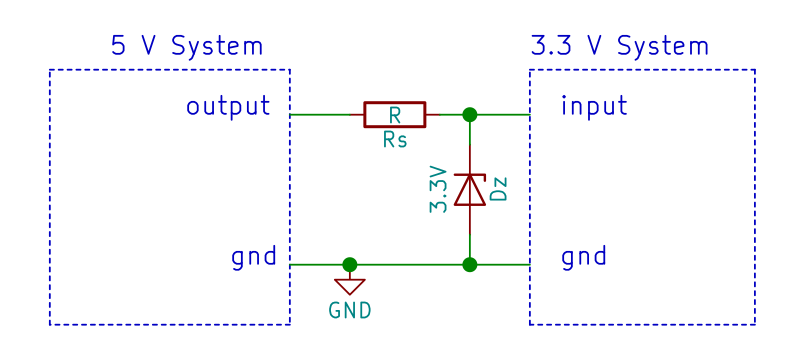Fig. 7. A 3.3V Zener diode allows clamping the voltage.

• Bandwidth similar to the Schottky or active clamp solution, due to the possibility of using low-value resistors.
• No current injected into the 3.3V supply line.
• No current injected into input pin.
• Lower cost than the BJT or Schottky.
• The clamping voltage can be lower than the BJT.

• Requires two components per input (resistor and zener).
• High power usage (with low-value resistors) or poor bandwidth (high value resistors).
• Output overload (with low-value resistors)
• The capacitance of the diode must be still taken into account.
• The output voltage is not precise, because some low-valued Zener diodes have a very poor dynamic resistance.
• The 3.3V input must have a high impedance (small input leakage, such as CMOS).

## SERIES DIODE WITH PULL-UP RESISTOR.

If your 3.3V system accept low-level input voltages as high as 0.7V, you can use a normal 1N4148 diode, as shown below. If you want more noise margin (or if simply 0.7V is too high), use a Schottky diode instead.

The working principle is simple: when the 5V system outputs 5V, the diode is reverse polarized, therefore it is open. The input of the 3.3V system is pulled up to 3.3V through R1. Then the 5V-system outputs 0V, the diode is forward biased, so the voltage at the 3.3V input will be the forward voltage drop of the diode: about 0.6-0.7V for silicon diodes, or 0.35V for Schottky diodes. Remember to use a fast signal diode! (i.e. do not use a 1N4007!).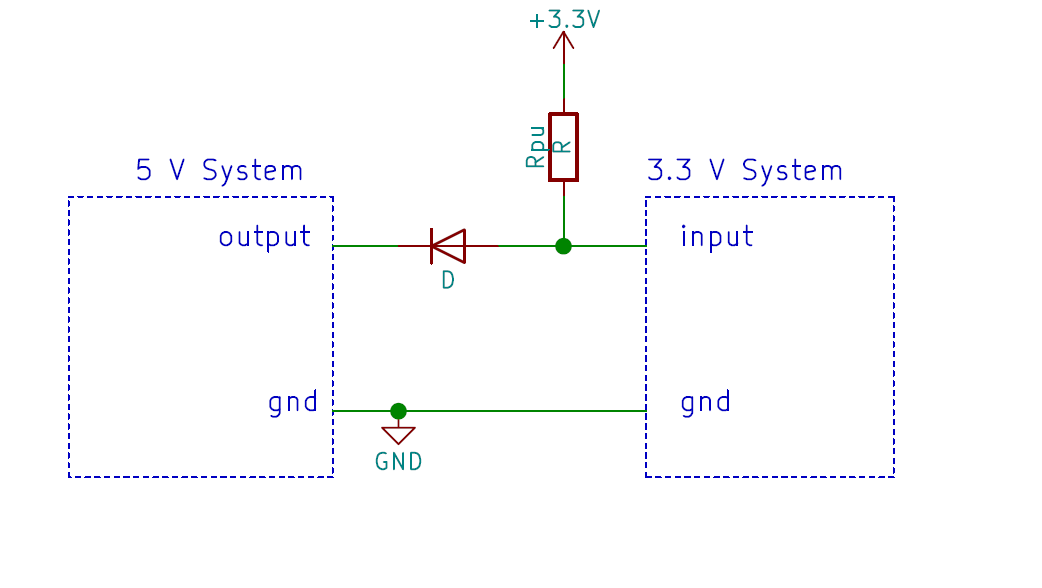Fig. 8. A series switching diode (such as 1N4148) blocks the high level voltage. The series resistor pulls-up the output.

• Bandwidth similar to the Schottky clamp solution, due to the possibility of using low-value pull-up resistors.
• No current injected into the 3.3V supply line.
• No current injected into input pin.

• Requires two components per input (resistor and diode).
• High power usage (with low-value resistors) or poor bandwidth (high value resistors).
• Output overload (with low-value resistors)
• The capacitance of the diode must be still taken into account.
• The low-level output voltage is the forward voltage drop of the diode.
• The 3.3V input must have a high impedance (small input leakage, such as CMOS).

## RESISTOR DIVIDER

One simple way to reduce the 5V voltage, is through a resistor divider!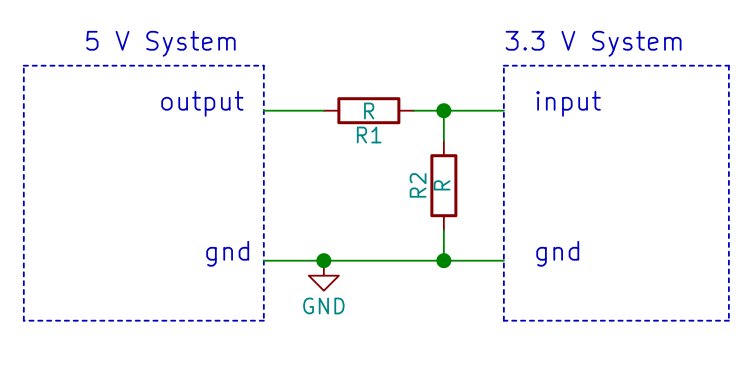Fig. 9. A resistor divider.

The resistor divider performs a better job, with respect the single resistor. Given the same bandwidth, it consumes less with respect to the other solutions already examinated (except the direct connections!).

In fact, the bandwidth in all the solutions is determined by the time constant RSCparasitic (note also that the BJT or the diodes increase Cparasitic!!!). The current is (5V-3.3V)/RS. On the resistor divider, the current is (5V-3.3V)/R1. However, the bandwidth of the divider is determined by the time constant (R1//R2 Cparasitic). In other words, if RS=R1, the bandwidth will be higher on the divider. Conversely, if the bandwidth is the same, the current will be smaller.

The parasitic capacity of R2 still impact on the bandwidth, but this is much less with respect to a diode or a BJT.

• Better bandwidth with respect to the other systems (except direct connection) at the same consumption (or smaller consumption at the same bandwidth).
• No current injected into the 3.3V supply line.
• No current injected into input pin.
• Lower cost than the BJT, Schottky, or zener.
• Symmetric rise/fall times.

• Requires two components per input (two resistors).
• High power usage (with low-value resistors) or poor bandwidth (high value resistor).
• Output overload (with low-value resistors)
• The 3.3V input must have a high impedance (small input leakage, such as CMOS).

# BJT/MOSFET AS INVERTERS

If you can live with inverted signals, you can use a BJT or MOSFET.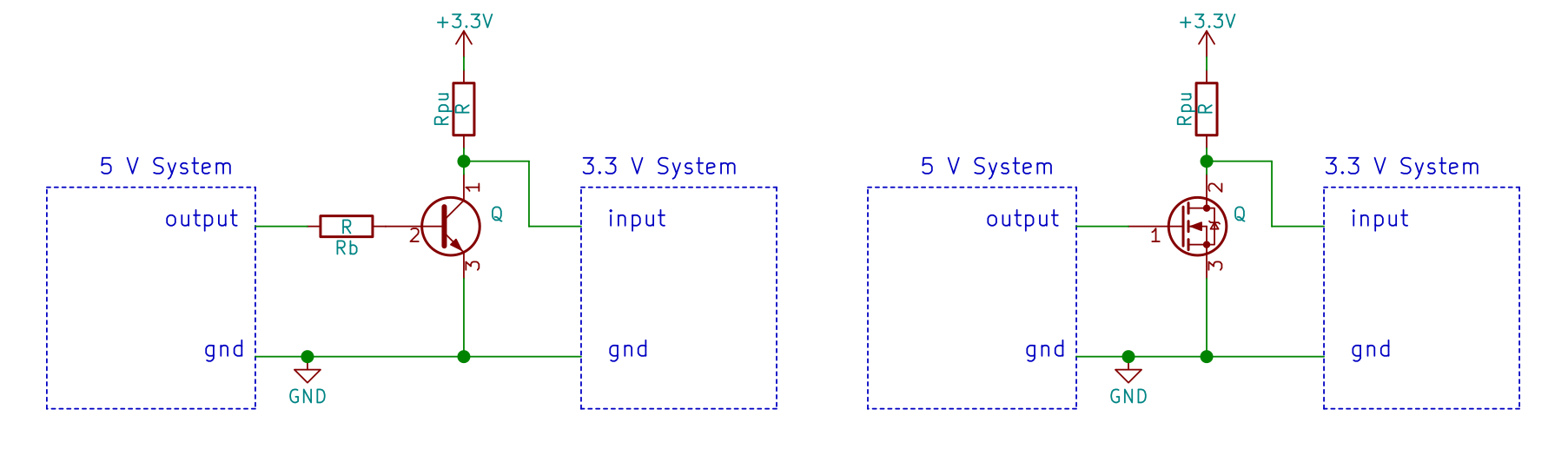Fig. 10. A BJT/MOSFET as inverter can be used as level shifter.

The bandwidth is determined mainly by the pull-up resistor and the parasitic capacitance (which must include the capacitance of the BJT/MOSFET!).

• No current injected into the 3.3V supply line.
• No current injected into input pin.

• Requires two or three components per input (the BJT/MOSFET and one or two resistors).
• High power usage (with low-value pull-up resistors)
• Inverted output!
• The 3.3V input must have a high impedance (small input leakage, such as CMOS).

# TWO CASCADED BJT/MOSFET AS BUFFER.

You can cascade two of the previous BJT/MOSFET-resistor inverters. In this way the output is not inverted.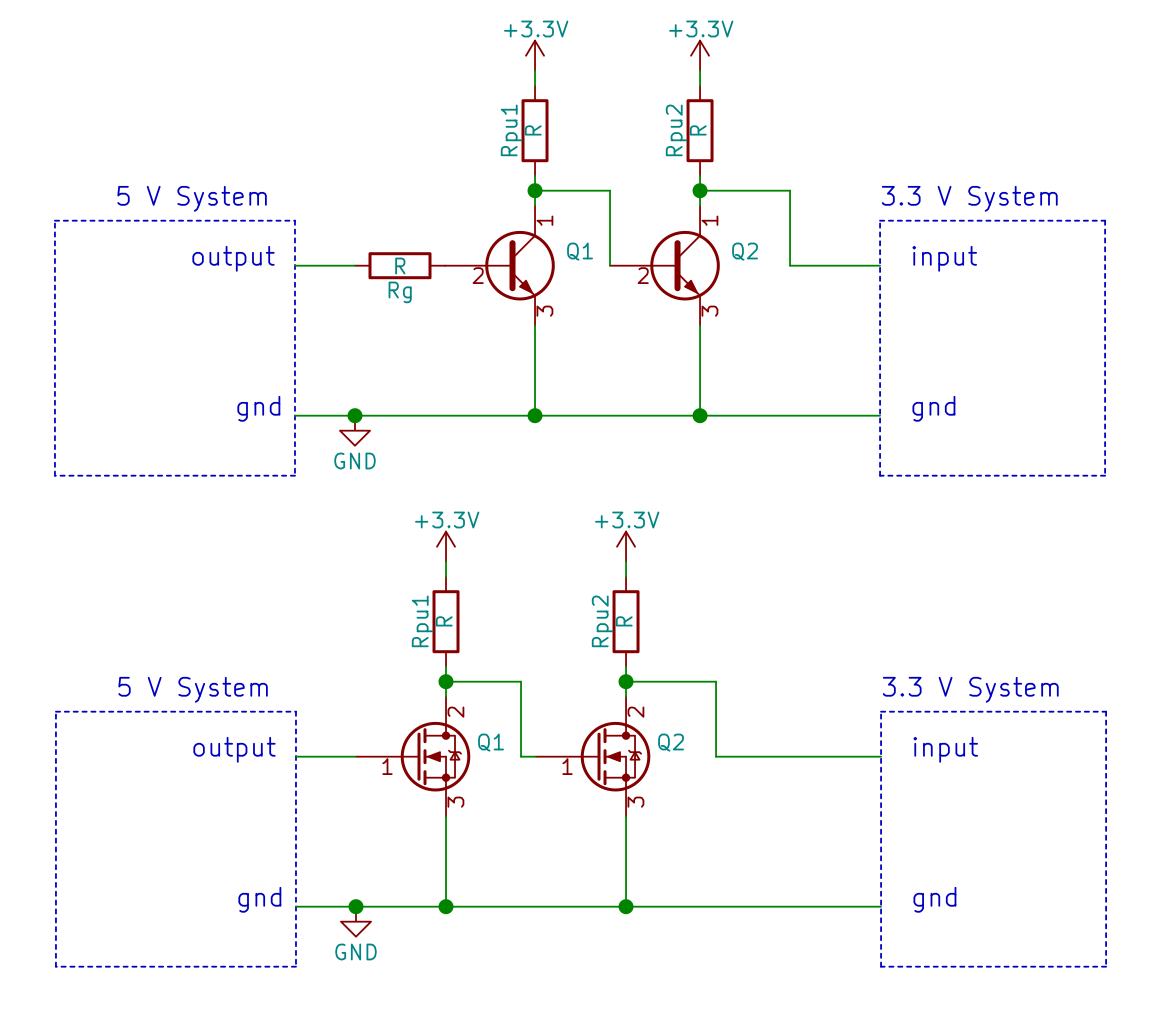Fig. 11. You can cascade two inverters made with a BJT or MOSFET and achieve a level shifter.

• No current injected into the 3.3V supply line.
• No current injected into input pin.
• The logic level is not inverted.

• Requires a lot of components!
• High power usage (with low-value pull-up resistors)
• The 3.3V input must have a high impedance (small input leakage, such as CMOS).
• Bandwidth smaller with respect to the previous case, because of the cascading of two stages.

# PULL-UP RESISTOR on OPEN DRAIN/COLLECOR OUTPUTs.

This WORKS ONLY if the output is an open collector/drain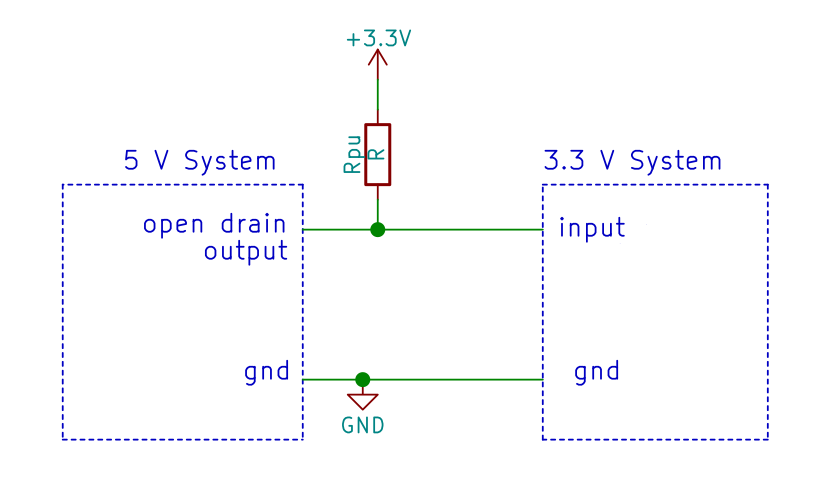Fig. 12. If the output is a open drain/collector, you can use a pull-up resistor.

This is extremely similar to the “BJT/MOSFET as inverter”. Since the output is open drain, a pull-up resistor must be inserted.

• Single resistor required.
• No current injected into pin.
• No current injected into the 3.3V line.

• High power usage (with low-value resistors) or poor bandwidth (high value resistors).
• The 3.3V input must have a high impedance (small input leakage, such as CMOS).

# LOGIC ICs (Buffer, gates, etc) WITH OPEN DRAIN/COLLECTOR OUTPUT.

You can connect open drain/collector buffer/gate IC, plus a pull up resistor as shown below. The buffer/gate is powered with the 5V, so that it will accept the 5V.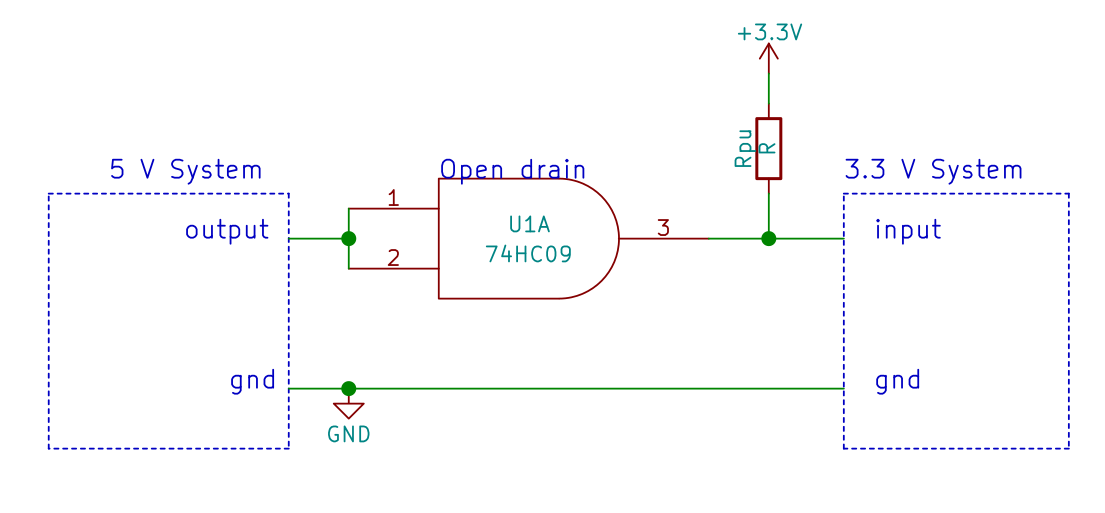Fig. 13. You can add a gate (e.g. 74HC09) or a buffer (e.g. 74LS07) powered at 5V, with an open drain output.

• No current injected into pin.
• No current injected into the 3.3V line.

• High power usage (with low-value resistors) or poor bandwidth (high value resistors).
• This solution requires a buffer and a resistor.
• The 3.3V input must have a high impedance (small input leakage, such as CMOS).

# ADDING 3.3V-POWERED LOGIC ICs (Buffer, gates, etc) WITH 5-V tolerant input.

You can connect a 5V tolerant IC, powered at 3.3V, such as SN74LV1T34.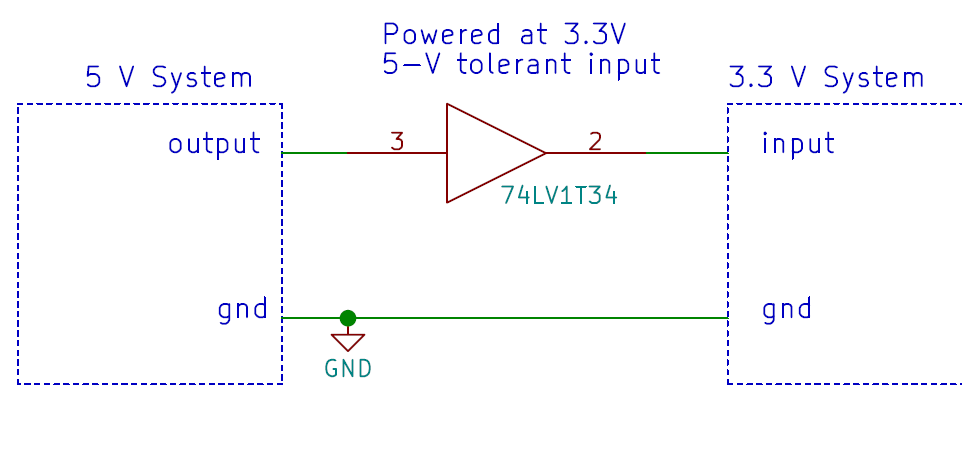Fig. 14. You can add a 5-V tolerant buffer (powered at 3.3V).

• No current injected into pin.
• No current injected into the 3.3V line.
• Low power.
• Fast.
• High noise margin.

• Cost and space

## SERIES MOSFET (common gate configuration)

Instead of using the MOSFET in common source configuration, we can use the commong gate configuration. The MOSFET will be actually in series. The MOSFET must be a 2.5V logic level MOSFET otherwise it won’t work.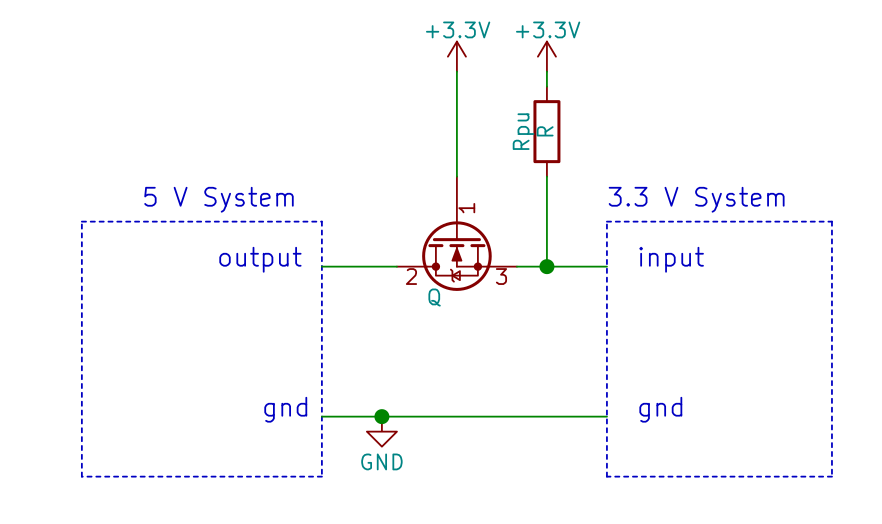Fig. 15. A series MOSFET can be used as translator.

The circuit works as follows.

When the VIN is at 5V, the MOSFET will be OFF, as VGS = 0V (tied up by R2). When VIN is 0V, the output will initially go to 0.7V, due to the drain-body parasitic diode of the MOSFET. Therefore VGS will become 3.3V-0.7V = 2.6V. Since this is a 2.5-V logic level MOSFET, the MOSFET will turn on, effectively acting now as a short. The output will be 0V.

As we will show in “Interfacing a 3.3V to 5V system”, this circuit works also bidirectionally, i.e. it translates a 3.3V signal into a 5V signal!

• No current injected into the 3.3V supply line.
• No current injected into input pin.
• Non-inverted output.
• Can be bidirectional.

• Requires two components per input (the MOSFET and one resistor).
• High power usage (with low-value pull-up resistors)
• Output overload (with low-value pull-up resistors)
• The capacitance of the MOSFET must be still taken into account.
• Requires a 2.5V logic level MOSFET.
• The 3.3V input must have a high impedance (small input leakage, such as CMOS).

## SERIES BJT (common base configuration)

A BJT can be also used, however the emitter must be on the 5V side (the source of the MOSFET was, instead, on the 3.3V side!).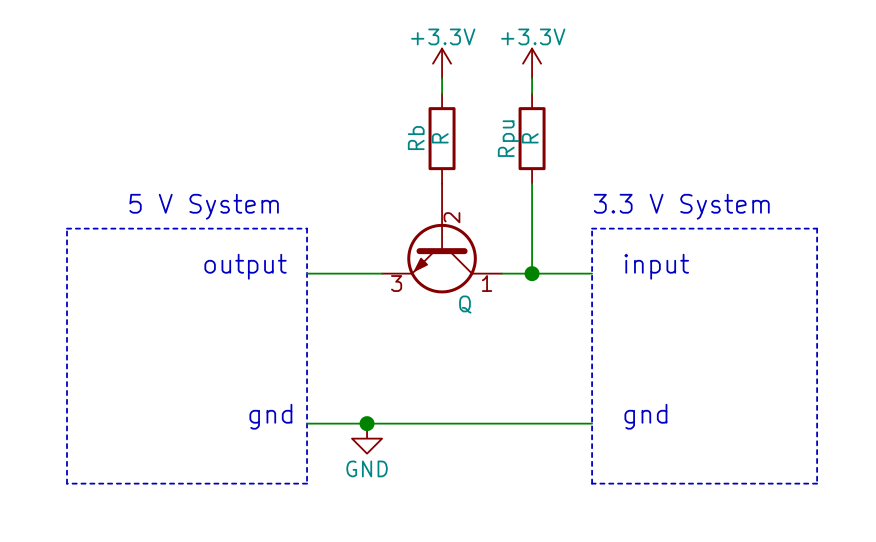Fig. 16. The BJT can be used as series translator as well!

It works as follows: when the input is 5V, VBE=-1.7, therefore the BJT is OFF. The 3.3V side is pulled-up. When the VIN is 0V, the base-emitter junction will be forward polarized, and the BJT will turn on, transmitting the “0” logic level to the 3.3V side.

• No current injected into the 3.3V supply line.
• No current injected into input pin.
• Non inverted output.

• Requires three components per input (the BJT and two resistors).
• High power usage (with low-value pull-up resistors)
• Output overload (with low-value pull-up resistors)
• The capacitance of the BJT must be still taken into account.
• VCE,sat is added to the low value output.
• The 3.3V input must have a high impedance (small input leakage, such as CMOS).

## LEVEL TRANSLATOR

To achieve the fastest speed, lowest power consumption and better signal levels, you can use a level translator IC such as 74LVC8T245 (8 bit) or 74LVC1T45 (1 bit). They however cost a lot, occupy space, and require other two decoupling capacitors (not shown in the schematics).

Usually level translators come with different number of inputs/outputs, they can be also bidirectional (either controlled by data direction, or automatic).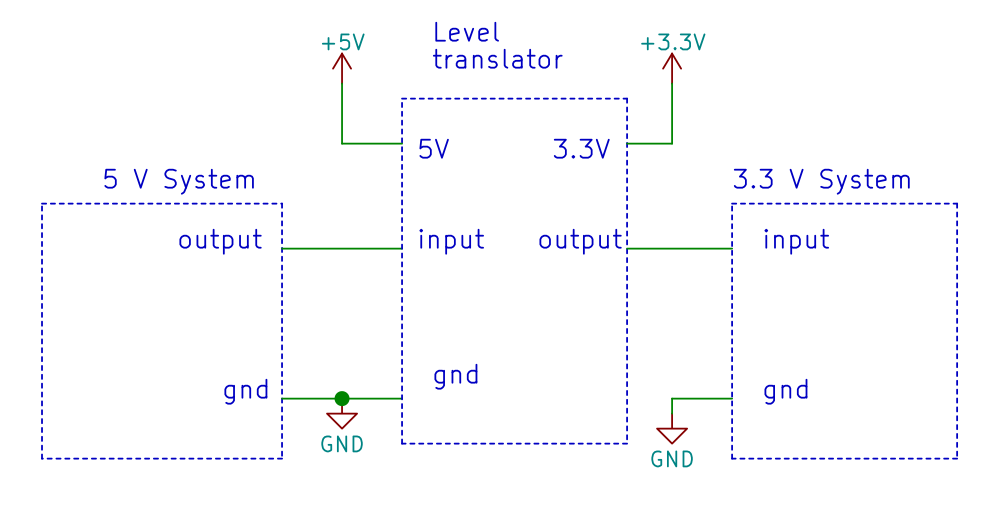Fig. 17. A level translator allows for the cleanest signal, with the best performance. However, it adds space and cost.

• No current injected into the 3.3V supply line.
• No current injected into input pin.
• Not inverted output (There are also inverting translators too).
• Very high bandwidth.
• Good signal levels.
• The output of the transceiver can be also loaded.
• Negligible static power consumption.

• Cost (level translators are much more expensive than a pair of resistors or a MOSFET/diode).
• Space required (especially with if few inputs are required).

## OPTOCOUPLER/ISOLATOR

This technique is especially useful and recommended when you need to interface two separate systems, or when you need electrical insulation between two parts of the same circuit/system. It is also very useful when dealing with voltages much higher than 5V (e.g. 24V).

Note that the two systems might share the same ground too, but, in this case you will lose the electrical insulation between them.

There are 4 variants, considering the inverting or non inverting configuration.

In the schematics below, we show a standard optocoupler, with a transistor output. There are optocouplers that already provide a digital, and, wtih them, in some cases Rpu is not needed (check if the output is open collector).

Recently, other kinds of isolators have been available. These are not optical based, but rather on capacitive, giant magnetoresistive or magnetic couplings. These are generally faster, but much more expensive.

In all these optocoupler/isolator, with a direct digital output, only the first version of both the inverting and non inverting configurations can be used (i.e. where the optocoupler is connected between the input of the 3.3V system and ground).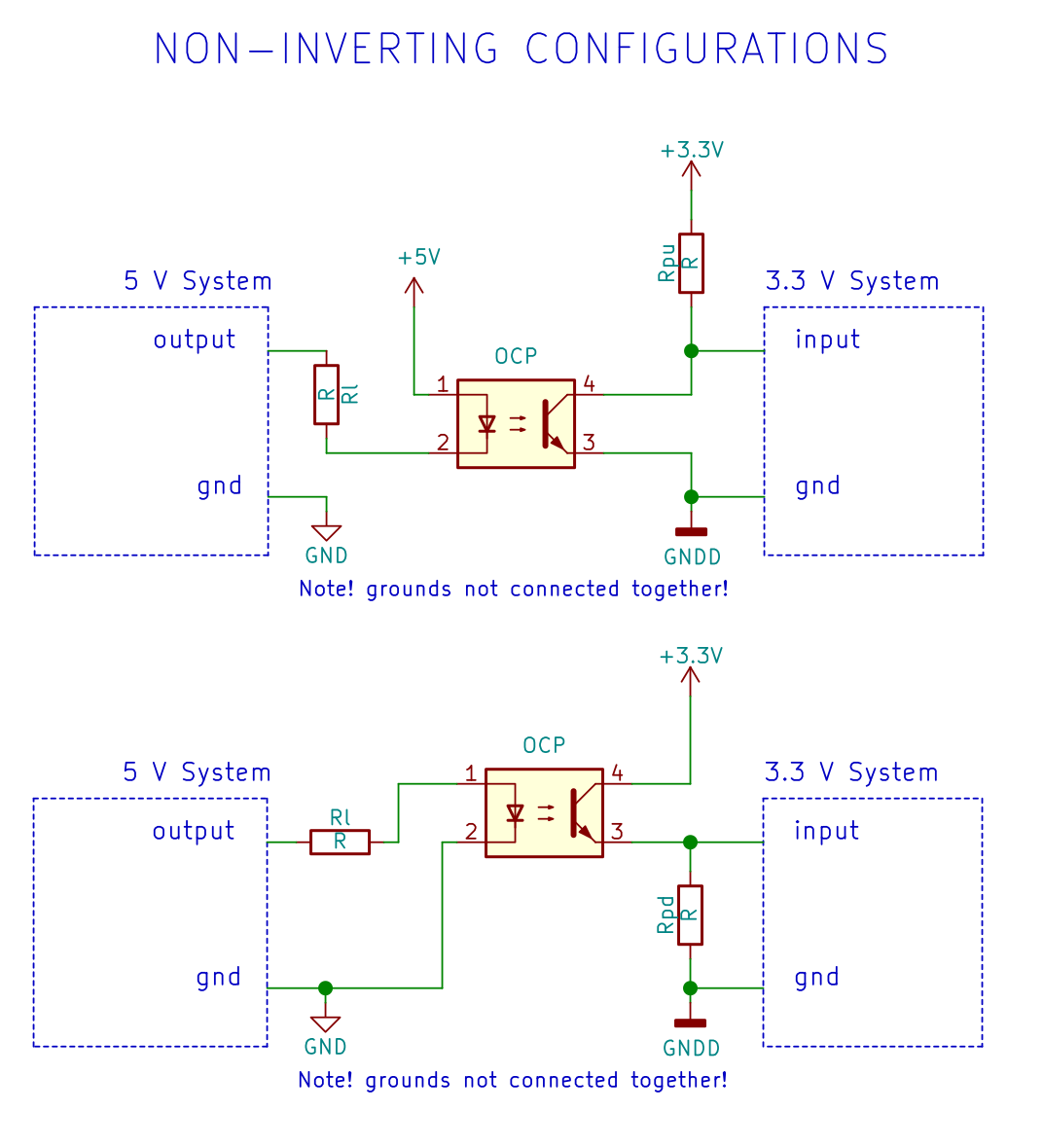Fig. 18. Two non inverting configurations using an optocoupler. In case of digital output, only the first choice is typically possible.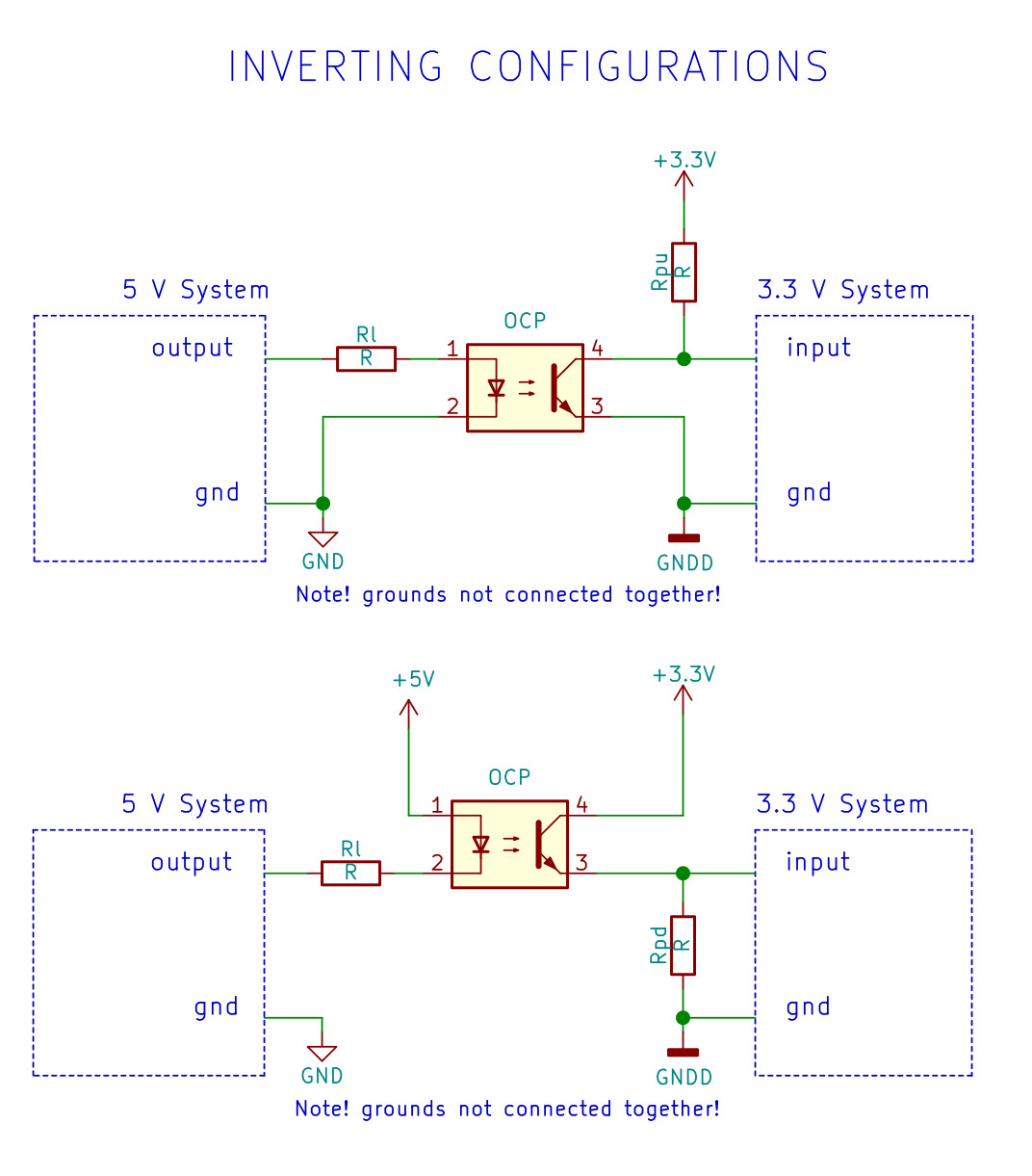Fig. 19. Two inverting configurations using an optocoupler. In case of digital output, only the first choice is typically possible.

• Electrical insulation between the two system. Not only it provides better noise performance, but also increases the safety of the system.
• Allows to convert any voltage level to “any” voltage level.
• By properly choosing the ground or power supply (depending on the configuration), you can achieve other kinds of voltage translation (e.g. -12V…+12V to 0…3.3V translation).

• It requires an optocoupler.
• Standard optocouplers are typically slow. For high data rates, special “high-speed” optocouplers are required.
• Relatively high current consumption due to the internal LED.
• The power consumption is higher if high bandwidth is required, as Rpu/Rpd need to be rather small, if small rise/fall times are required.

# Conclusions

These are just some of the many possibilities.

We will be adding them as soon as they come into our mind :).

1.Alan

Liked the article giving many good practical approached. But I fail to understand why a simple 3V3 voltage regulator cannot/ is not used. I must be missing something.

Do help me out pse.

1.N W (Post author)

Hi Alan!

We are glad you liked the post.

A 3.3V voltage regulator is used to provide the power supply for the 3.3V chips, such as logic ICs, microcontrollers, analog stuff etc. However, it cannot be used* to convert a 5V binary signal, which can be 5V or 0V, to a 3.3V one.

*well, in principle one could use a linear regulator to convert a 5V digital signal – provided that the 5V logic output can source enough current – to a 3V3 one**. However this would be a very inefficient and expensive way. For instance many regulator are simply too slow, or they can oscillate in absence of input and output capacitors. Such capacitors would further impact the speed – down to some kHz- and the dynamic power consumption. At this point a single high valued resistor would provide a much better solution.

** you would still require a rather low valued pull down resistor, to create a 0 logic level. This is because most of the linear regulator simply consist of a series PNP (LDO) or NPN transistor, so there is “almost nothing” (expect the resistor divider feedback network, which might be extremely high in low power devices) to “pull down” the output.

2.Dang. I thought I was good. Quite the list of tricks. Props.

3.yan

Excellent page, It is quite difficult to find comprehensive Discrete circuit comparison on the web like this.. Seems that some of the simplier solutions are even better than the most evolved ones (With FET/BJT). Maybe something to highligh

4.Vassilis

Can you suggest one of these circuits (or another one) to interface ANY voltage in the region of 5V to 24V? (I mean, 5-24V must be translated to 3.3V and 0V to 0V).

1.N W (Post author)

Hi Vassilis, we suggest in this case an optocoupler! We will add the corresponding circuit shortly!

This site uses Akismet to reduce spam. Learn how your comment data is processed.